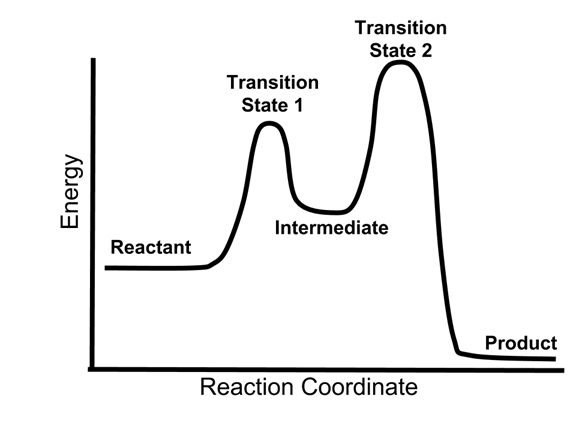# Enduring Understanding 4.C.1: Multistep Reactions

• Some reactions occur through a series of elementary steps, which add up to the overall reaction.
• The rate of a multistep reaction depends on what species are involved before the slowest (rate-determining) step.
• Species can be formed, then consumed, in the reaction, but do not exist for a long period of time and do not appear in the overall reaction equation. These transient species are called intermediates.
• The exact mechanism by which a multistep reaction occurs can be determined by observations such as
• Direct evidence of an intermediate
• The rate law by which the reaction proceeds.

• The energy diagram of a two-step reaction is shown below.

•• In the above reaction, a reactant goes through one elementary step with a lower activation energy (transition state 1) to form the intermediate. The intermediate then goes through a second step (transition state 2) with the highest energy barrier to form the product.
• Because the energy barrier is higher for the second step (transition state 2), this second step will be the rate-determining (slow) step.

• Example 1: The reaction of carbon monoxide and nitrogen dioxide:
• CO(g) +NO2 (g) → CO2 (g) + NO(g)

• Is a two step reaction, consisting of the following steps:
• 2 NO2 (g) → NO3(g) + NO(g)
NO3(g) + CO(g) → CO2 (g) + NO2(g)
• If the rate law of the reaction is given by rate = k[NO2]2, which step is the rate determining one?
• Reacting species that come before the rate determining elementary step will contribute to the rate.
• Since 2 NO2 molecules participate in the first step to form the intermediate NO3, and a CO molecule participates in the second, if the second step was the slow one, we would expect CO to be a part of the rate law.
• The conclusion is that the first step is the slow one, and the second step is fast:
• 2 NO2 (g) → NO3(g) + NO(g) (slow)
NO3(g) + CO(g) → CO2 (g) + NO2(g) (fast)

• Example 2: Given the following two step reaction:
• 2 NO (g) → N2O2(g) (slow)
N2O2(g) + O2 → 2NO2(g) (fast)
• Which is the most likely rate law for the reaction: k[NO]2, or k[NO]2[O2]
• The answer is k[NO]2, since 2 molecules of NO (and no O2) are involved in the reaction prior to the slow step.

 Related Links: Chemistry Chemistry Quizzes AP Chemistry Notes Boltzmann & Arrhenius

To link to this Multistep Reactions page, copy the following code to your site: